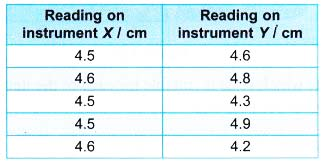# Understanding Measurements

## Understanding Measurements

1. Physics is based on measurements. Experiments which are the key to the development of physical theories involve measurements of various physical quantities.
2. Various measuring instruments are used for different types of measurements and the reading obtained by each instrument must be presented in suitable units.
3. Choosing an appropriate instrument to measure a physical quantity is important to ensure that the measurement is accurate and reproducible.
4. Consistency, accuracy and sensitivity are three important aspects of a measurement.

### Consistency and Accuracy

1. No measurement is exact. Every measurement is an estimation of the actual value. For example, even the most accurate clock used in the Olympics does not give the exact time of a 100 m runner. It only gives an accurate estimate of the actual time.2. Consistency and accuracy are two important properties of measurements. Consider a target as in Figure.
(a) The bullseye in the centre of the target represents the actual or true value of the quantity to be measured.
(b) Individual measurements of the quantity under identical conditions are represented schematically by individual shots taken by the marksman on the target.

(c) When the shots are closely clustered together, it shows that repeated measurements yield almost the same reading. We say that the measurements are consistent. Consistency of an instrument is the ability of the instrument to measure a quantity with little or no deviation among measurements.
Language support:
Deviation means the difference between the measured value and its mean value.

(d) The nearer a shot is to the bullseye, the nearer is the measurement to the actual value. We say that the measurement is accurate. Accuracy of a measurement is how close the measurement made to the actual value.

Example 1. Figure shows four sets of measurements on boards A, B, C and D each of which represent a rifle target.(a) Comment on the consistency and accuracy of the measurements of boards A, B and C.
(b) Suggest one possible reason for the set of measurements shown on board D.
Solution:
(a) Board A – Consistent and accurate
Board B – Consistent but not accurate
Board C – Not consistent and not accurate
(b) Three measurements are accurate and consistent. The other measurement may be due to a wrong reading.

Example 2. Instrument X and instrument Y are used to measure the thickness of a dictionary. Table shows two sets of readings taken by the respective instruments.Which of the two instruments is more consistent? Explain your answer.
Solution:
Instrument X is more consistent. This is because the measurements taken by instrument X are closer to each other (or there is little deviation between the measurements).

### Sensitivity of Measuring Instruments

1. Sensitivity of a measuring instrument is its ability to detect quickly a small change in the value of quantity to be measured.
2. The smaller the minimum scale division of a measuring instrument, the more sensitive is the instrument.
3. Table shows the sensitivity of some common instruments for measuring length.Example 3. A hawker has two measuring scales, A and B. A has a measuring range of 0 – 400 g and gives the smallest reading of 10 g or 0.01 kg. B has a measuring range of 0 – 4 kg and gives the smallest reading of 0.1 kg. A lady wants to buy a whole chicken of about 1.5 kg and red chillies of about 200 g.State which scale is suitable for each of the items and explain your answer.
Solution:
Scale B is more suitable for measuring a whole chicken because the mass of the chicken is out of the range of measurement of scale A.
Scale A is more suitable for measuring the red chillies because it is more sensitive and can detect small changes in the mass.

Example 4. A, B, C and D show the scales of four different meters respectively. Which meter is the most sensitive?Answer: A
Meter A has the smallest minimum scale division out of the four meters.Voltmeter A is more sensitive than voltmeter B because:

1. Volmeter A has a smaller minimum scale division compared to voltmeter B.
2. Voltmeter A can detect a smaller change in the voltage than voltmeter B.
3. For the same variation of voltage, voltmeter A gives a bigger deflection than voltmeter B.